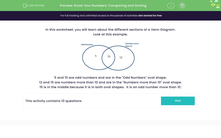# Know Your Numbers: Comparing and Sorting

In this worksheet, students compare and sort numbers into different categories.Key stage:  KS 1

Curriculum topic:   Number: Number and Place Value

Curriculum subtopic:   Order/Compare Numbers to 100

Difficulty level:#### Worksheet Overview

In this worksheet, you will learn about the different sections of a Venn Diagram.

Look at this example.5 and 15 are odd numbers and are in the "Odd Numbers" oval shape.

12 and 15 are numbers more than 10 and are in the "Numbers more than 10" oval shape.

15 is in the middle because it is in both oval shapes.  It is an odd number more than 10.

Let's have a look at another example:

Choose the right section for the number 13.Let's start by looking at the categories we have here: numbers more than 10 and odd numbers.

We have the find the right place for 13.

Is 13 an odd number? Yes.

Is 13 bigger than 10? Yes.

As it is in both categories it will go in the middle group as this is where both categories cross over.

This means 13 will go in B.

### What is EdPlace?

We're your National Curriculum aligned online education content provider helping each child succeed in English, maths and science from year 1 to GCSE. With an EdPlace account you’ll be able to track and measure progress, helping each child achieve their best. We build confidence and attainment by personalising each child’s learning at a level that suits them.

Get started